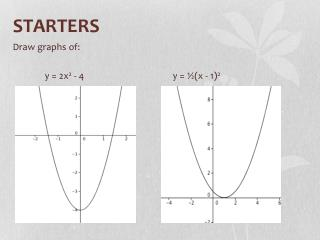DownloadDownload PresentationSTARTERS

# STARTERS

Download Presentation## STARTERS

- - - - - - - - - - - - - - - - - - - - - - - - - - - E N D - - - - - - - - - - - - - - - - - - - - - - - - - - -
##### Presentation Transcript

1. STARTERS Draw graphs of: y = 2x2 - 4 y = ½(x - 1)2

2. Note 6: Finding Equations of Parabolas • Use the key points of parabolas to write equations: • x and y intercepts • axis of symmetry • vertex

3. Examples: Find the equations for the following graphs y-intercept = 2 Equation: y = x2 + 2

4. Examples: Find the equations for the following graphs x-intercepts -2 and 3 Equation: y = (x + 2)(x – 3)

5. Examples: Find the equations for the following graphs vertex (2, 3) Equation: y = (x – 2)2 + 3

6. Graphics Calculator instructions • Use the stats function to find equations. • Stats Function • Delete any data in columns: (F6) DELA (F4), YES (F1) • Enter co-ordinates: (X – List 1, Y – List 2) • GRPH (F1) • SET (F6): Graph type Scatter • X List List 1 • Y List List 2 EXIT • Graph points: GPH1 • Draw line: CALC (F1), x^2 (F4) – Parabola

7. Page 55 Questions 113 - 114

8. If the parabola is not the usual pattern of across 1 up 1, then use algebra to solve for the scale factor. Worksheet